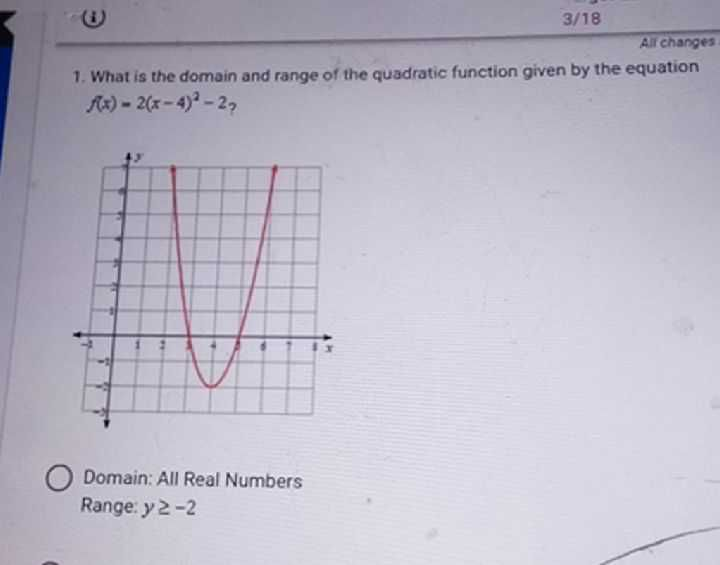### ¿Todavía tienes preguntas de matemáticas?

Pregunte a nuestros tutores expertos
Algebra
Pregunta1. What is the domain and range of the quadratic function given by the equation

$$f ( x ) = 2 ( x - 4 ) ^ { 2 } - 2$$ ?

Domain: All Real Numbers

Range: $$y \geq - 2$$

Range: $$y \geq - 2$$